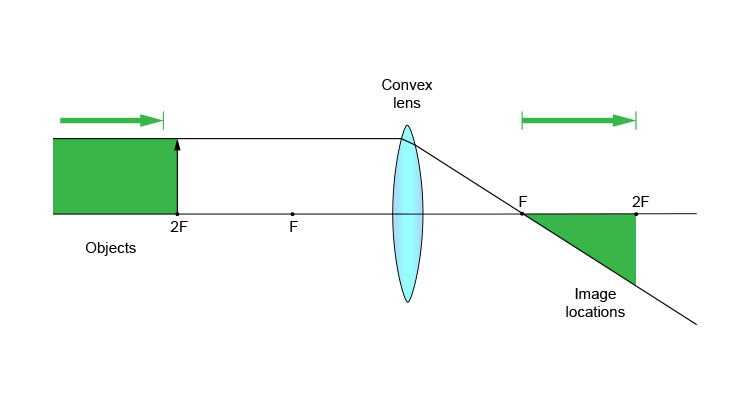# Convex lens – object at more than 2F

This means that the object is further away than two principal focal lengths from the convex lens.

The ray diagram below shows you where the image will be located using the two rules:

1. From the object, pass a parallel line through the principal focal point.
2. From the object, pass a ray line through the centre of the convex lens.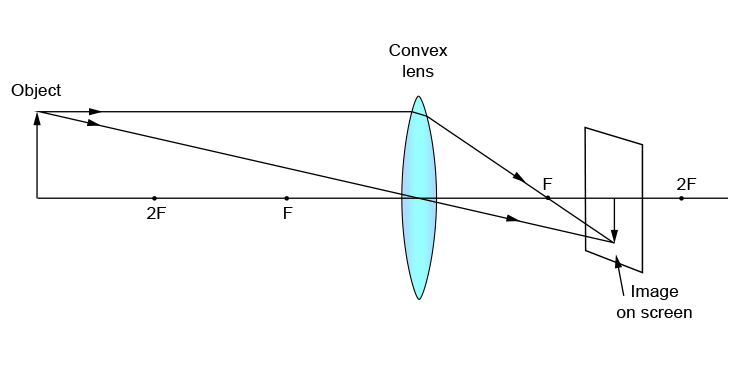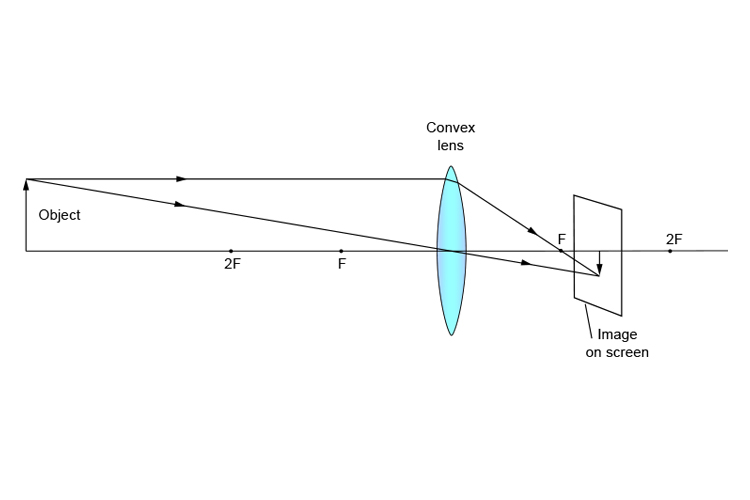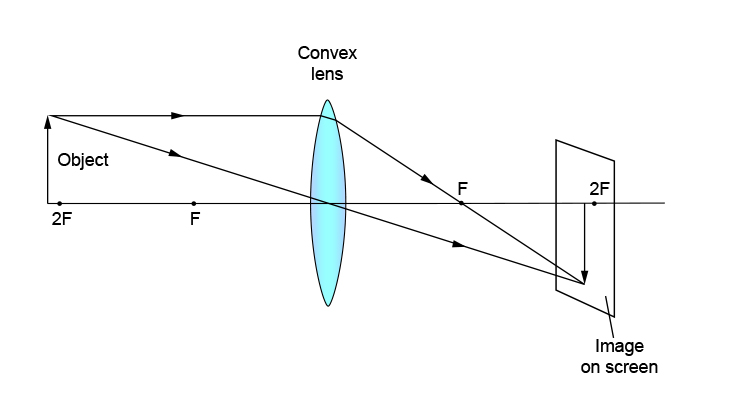We can observe from these ray diagrams that any object more than two principal focal lengths (more than 2F) from the lens will always be located between one principal focal length and two principal focal lengths (between 1F and 2F) on the opposite side of the lens. The image will always be inverted and smaller. All these images can be captured on a screen so they must be real images.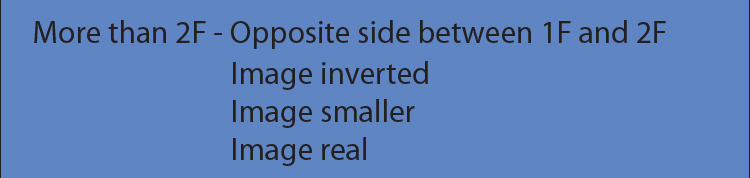In summary this would be: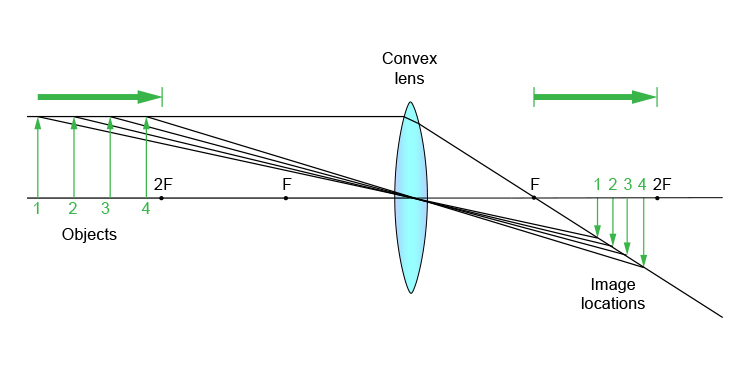Or to make it really simple: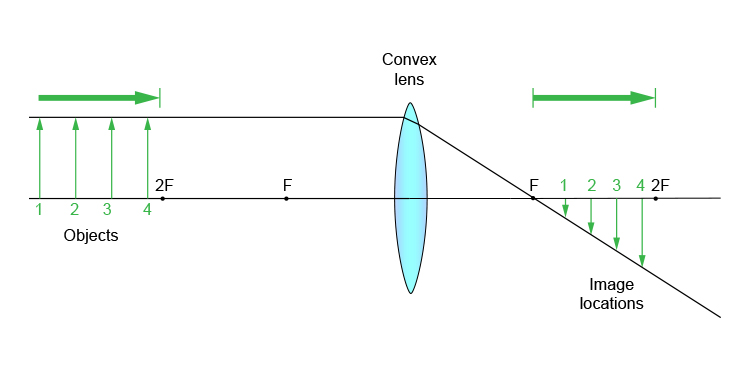Or as a block diagram